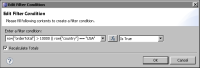Creating multiple filter conditions
The filter tool supports the creation of any number of conditions for filtering data. BIRT evaluates each condition and includes only data rows that meet all the conditions. For example, assume the following two conditions were created with the filter tool:
row["orderTotal"] Larger Than 10000
row["country"] Equal "USA"
In this example, BIRT includes a row only if the value in the orderTotal field is greater than 10000 and the value in the country field is equal to USA. In other words, creating two filter conditions is equivalent to specifying the following JavaScript expression:
row["orderTotal"] > 10000 && row["country"] == "USA"
The following rows meet the specified filter conditions:
Country Order ID Order Total

USA 1001 12000
USA 1010 15000
USA 1035 18500
USA 1155 25000
USA 1200 12000
USA 1455 20500
To return a row if it meets any one of multiple conditions, create a single filter condition that uses the OR (||) operator to combine multiple conditions. For example, to include a row where either orderTotal exceeds 10000 or country is USA, create an expression that compares to true, as follows:
row["orderTotal"] > 10000 || row["country"] == "USA" Is True
For expressions that compare to true or false, you must use the comparison operator. As the previous example shows, use ==, not the assignment operator, =. Figure 12‑16 shows how the filter condition appears in the filter tool. In the first field, instead of selecting a field from the drop-down list, open the expression builder and type the expression. Then, in the second field, select the Is True operator.Figure 12‑16 Filter condition that uses the OR and Is True operators
In this example, the following rows meet the specified filter condition:
Country Order ID Order Total

Belgium 1020 21000
France 2005 14500
USA 1425 5000
USA 1750 7500
USA 1001 12000
USA 1010 15000
USA 1035 18500
USA 1155 25000
USA 1200 12000
USA 1455 20500